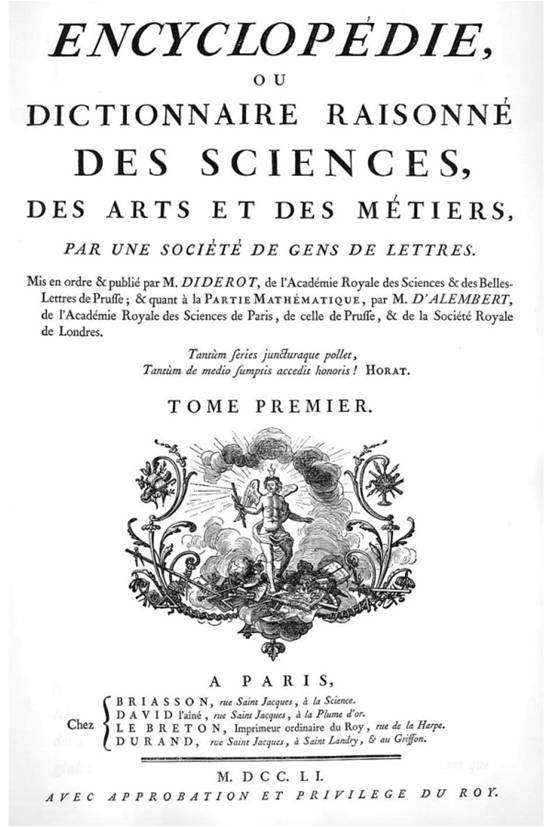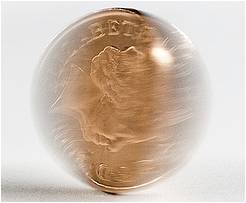# A Brief History of Probability

##### Age 11 to 14 Challenge Level:

This activity was developed by the epiSTEMe project. More information about the project, and the other epiSTEMe activities available on NRICH, can be found here.

## The Probability of at Least One Head in Two Flips of a Coin

This activity has three main purposes:
• To convey an idea of the historical origins of probability.
• To deconstruct common misconceptions about combined trials.
• To introduce the idea of systematically identifying combined outcomes.

### ActivityIn the mid eighteenth century a craze for games of chance was sweeping across Europe.

Analysing such games inspired the new science of probability.

In this period, too, leading thinkers were working on a new kind of book - an encylopædia.

The French mathematician, d’Alembert, was asked to write a chapter on probability.

He had to decide what to say about probabilities still being debated at the time, such as:

What is the probability of at least one Head in two flips of a coin?
Consider the three possible arguments below:A
These are the combinations possible for two coin flips:
Tail & Tail
3 of these 4 cases include at least one Head.
So the probability is $\tfrac{3}{4}$.

B
The probability of a Head on the first flip is $\tfrac 12$.
The probability of a Head on the second flip is another $\tfrac 12$.
So the combined probability of at least one Head is 1.

C
If the first flip gives a Head, then another flip isn’t needed.
If a second flip is needed it will either produce a Head or not.
2 of these 3 cases result in at least one Head.
So the probability is $\tfrac 23$.

Which of these arguments should d’Alembert accept?
Should he add some further explanation when he writes his chapter?

These are available as slides for display, in PowerPoint or PDF formats.

As a whole class, discuss the information about D'Alembert. Then preview the probability

Organise the students into groups. Remind them of the ground rules for discussion.
Tell them they must decide and record whether they agree or disagree with each solution.

Whilst the groups are working, circulate to gain information about the thinking within different groups.

Invite one group to speak in support of the solution they have agreed to accept.

Invite further groups to speak in support of other solutions. Structure collective exploration of differences. If there continues to be disagreement, identify any students who have changed their mind, and ask them to explain the reasons why.

Should all the groups have agreed on the same solution, invite reasons for not choosing each of the other solutions.

Conclude by telling students that d’Alembert found it very hard indeed to decide between two of the solutions. He knew that people tended to agree with A, but he was still attracted to C. So he included both in his encyclopedia chapter!

### Key Points

Solution A identifies the combined outcomes of two flips of a coin in a systematic way (although it does not make it explicit that these outcomes are equally likely). Solution B implies that at least one head is a certainty. Solution C identifies three distinct outcomes or events in a systematic way but they are not equally likely: in particular, ‘Head on first flip’ has a probability of ½; which can be shown by comparing A and C as follows:

 Solution A Solution C Head & Head Head on first flip Head & Tail Tail & Head Tail on first flip followed by Head on second flip Tail & Tail Tail on first flip followed by Tail on second flip